Algebra

Equations

Inequalities

Graphs

Numbers

Calculus

Matrices

Tutorials

Enter an expression and click the Simplify button.

# Simplifying Polynomials

In section 3 of chapter 1 there are several very important definitions, which we have used many times. Since these definitions take on new importance in this chapter, we will repeat them.

When an algebraic expression is composed of parts connected by + or - signs, these parts, along with their signs, are called the terms of the expression.

a + b has two terms.
2x + 5y - 3 has three terms.

 In a + b the terms are a and b. In 2x + 5y - 3 the terms are 2x, 5y, and -3.

When an algebraic expression is composed of parts to be multiplied, these parts are called the factors of the expression.

ab has factors, a and b.

It is very important to be able to distinguish between terms and factors. Rules that apply to terms will not, in general, apply to factors. When naming terms or factors, it is necessary to regard the entire expression.

 From now on through all algebra you will be using the words term and factor. Make sure you understand the definitions.

An exponent is a numeral used to indicate how many times a factor is to be used in a product. An exponent is usually written as a smaller (in size) numeral slightly above and to the right of the factor affected by the exponent.

 An exponent is sometimes referred to as a "power." For example, 53 could be referred to as "five to the third power."

Note the difference between 2x3 and (2x)3. From using parentheses as grouping symbols we see that

2x3 means 2(x)(x)(x), whereas (2x)3 means (2x)(2x)(2x) or 8x3.

 Unless parentheses are used, the exponent only affects the factor directly preceding it.

In an expression such as 5x4
5 is the coefficient,
x is the base,
4 is the exponent.
5x4 means 5(x)(x)(x)(x).

Note that only the base is affected by the exponent.

 Many students make the error of multiplying the base by the exponent.For example, they will say 34 = 12 instead of the correct answer, 34 = (3)(3)(3)(3) = 81.

When we write a literal number such as x, it will be understood that the coefficient is one and the exponent is one. This can be very important in many operations.

x means 1x1.

 It is also understood that a written numeral such as 3 has an exponent of 1. We just do not bother to write an exponent of 1.

## MULTIPLICATION LAW OF EXPONENTS

### OBJECTIVES

Upon completing this section you should be able to correctly apply the first law of exponents.

Now that we have reviewed these definitions we wish to establish the very important laws of exponents. These laws are derived directly from the definitions.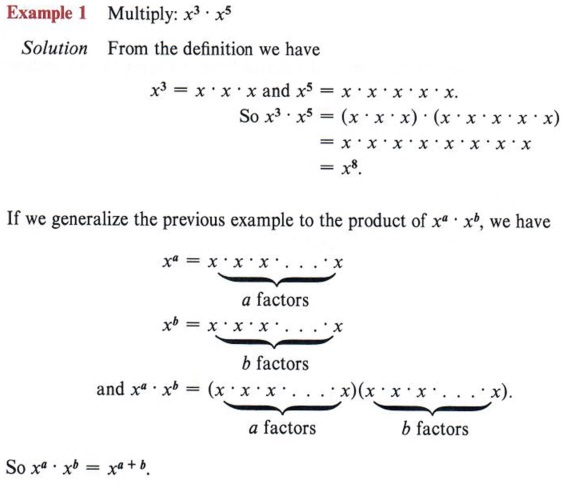First Law of Exponents If a and b are positive integers and x is a real number, then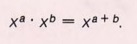To multiply factors having the same base add the exponents.

For any rule, law, or formula we must always be very careful to meet the conditions required before attempting to apply it. Note in the above law that the base is the same in both factors. This law applies only when this condition is met.These factors do not have the same base.

An exponent of 1 is not usually written. When we write x, the exponent is assumed: x = x1. This fact is necessary to apply the laws of exponents.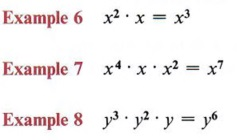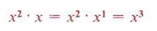If an expression contains the product of different bases, we apply the law to those bases that are alike.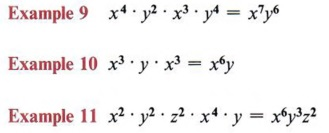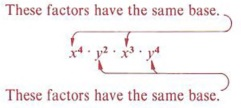## MULTIPLICATION OF MONOMIALS

### OBJECTIVES

Upon completing this section you should be able to:

1. Recognize a monomial.
2. Find the product of several monomials.

A monomial is an algebraic expression in which the literal numbers are related only by the operation of multiplication.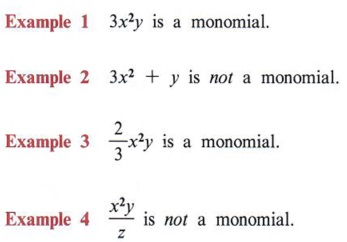is not a monomial since the operation of addition is involved.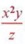involves the operation of division.

To find the product of two monomials multiply the numerical coefficients and apply the first law of exponents to the literal factors.

 Do you remember the first law of exponents?Multiply 5 times 3 and add the exponents of x. Remember, if an exponent is not written, an exponent of one is understood.

## MONOMIALS MULTIPLIED BY POLYNOMIALS

### OBJECTIVES

Upon completing this section you should be able to:

1. Recognize polynomials.
2. Identify binomials and trinomials.
3. Find the product of a monomial and binomial.

A polynomial is the sum or difference of one or more monomials.Generally, if there is more than one variable, a polynomial is written in alphabetical order.

Special names are used for some polynomials. If a polynomial has two terms it is called a binomial.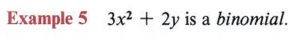If a polynomial has three terms it is called a trinomial.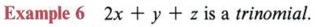In the process of removing parentheses we have already noted that all terms in the parentheses are affected by the sign or number preceding the parentheses. We now extend this idea to multiply a monomial by a polynomial.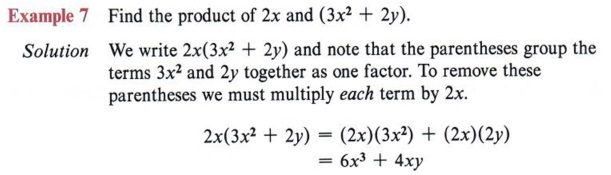Placing 2x directly in front of the parentheses means to multiply the expression in the parentheses by 2x. Notice each term is multiplied by 2x.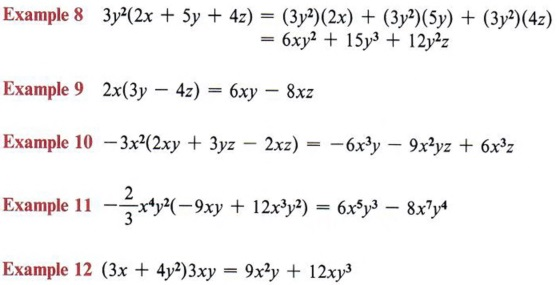Again, each term in the parentheses is multiplied by 3y2

 Again, each term in the parentheses is multiplied by 3y2. In each of these examples we are using the distributive property.

## PRODUCTS OF POLYNOMIALS

### OBJECTIVES

Upon completing this section you should be able to:

1. Find the product of two binomials.
2. Use the distributive property to multiply any two polynomials.

In the previous section you learned that the product A(2x + y) expands to A(2x) + A(y).

Now consider the product (3x + z)(2x + y).

Since (3x + z) is in parentheses, we can treat it as a single factor and expand (3x + z)(2x + y) in the same manner as A(2x + y). This gives usIf we now expand each of these terms, we haveNotice that in the final answer each term of one parentheses is multiplied by every term of the other parentheses.

 Note that this is an application of the distributive property.Note that this is an application of the distributive property.

Since - 8x and 15x are similar terms, we may combine them to obtain 7x.

In this example we were able to combine two of the terms to simplify the final answer.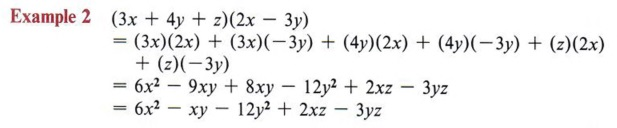Here again we combined some terms to simplify the final answer. Note that the order of terms in the final answer does not affect the correctness of the solution.

 The commutative property allows the rearrangement of order.Try to establish a system for multiplying each term of one parentheses by each term of the other. In these examples we have taken the first term in the first set of parentheses and multiplied it by each term in the second set of parentheses. Then we took the second term of the first set and multiplied it by each term of the second set, and so on.

## POWERS OF POWERS AND SQUARE ROOTS

### OBJECTIVES

Upon completing this section you should be able to:

1. Correctly apply the second law of exponents.
2. Find the square roots and principal square roots of numbers that are perfect squares.

We now wish to establish a second law of exponents. Note in the following examples how this law is derived by using the definition of an exponent and the first law of exponents.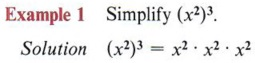by the meaning of the exponent 3.

Now by the first law of exponents we have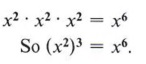In general, we note that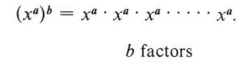This means that the answer will be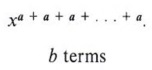Remember, to multiply common bases add the exponents.If we sum the term a b times, we have the product of a and b. Hence we see that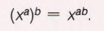Second Law of Exponents If a and b are positive integers and x is a real number, then.

In words, "to raise a power of the base x to a power, multiply the exponents."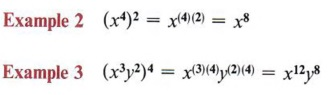.

 Note that each exponent must be multiplied by 4.

Note that when factors are grouped in parentheses, each factor is affected by the exponent.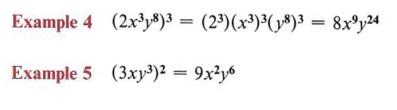.

Again, each factor must be raised to the third power.

Using the definition of exponents, (5)2 = 25. We say that 25 is the square of 5. We now introduce a new term in our algebraic language. If 25 is the square of 5, then 5 is said to be a square root of 25.

If x2 = y, then x is a square root of y.

 Notice, we say 5 is a square root and not the square root. You will see why shortly.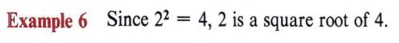.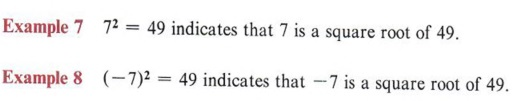From the last two examples you will note that 49 has two square roots, 7 and - 7. It is true, in fact, that every positive number has two square roots.

 In fact, one square root is positive and the other negative.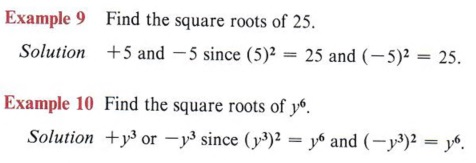.

 What are the square roots of 36?

The principal square root of a positive number is the positive square root.

The symbol "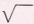" is called a radical sign and indicates the principal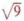indicates the principal square root or positive square root of 9.

Note the difference in these two problems.

a. Find the square roots of 25.
b. Find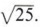.

 It is very important that you understand the difference between these two statements.

For a. the answer is +5 and -5 since ( + 5)2 = 25 and ( - 5)2 = 25.
For b. the answer is +5 since the radical sign represents the principal or positive square root.
Whole numbers such as 16, 25, 36, and so on, whose square roots are integers, are called perfect square numbers. For the present time we are interested only in square roots of perfect square numbers. In a later chapter we will deal with estimating and simplifying the indicated square root of numbers that are not perfect square numbers.

 You may sometimes see the symbol +/-. This means both square roots of a number are called for. For example,+/- 5 is the short way of writing + 5 and -5.

## THE DIVISION LAW OF EXPONENTS

### OBJECTIVES

Upon completing this section you should be able to correctly apply the third law of exponents.

Before proceeding to establish the third law of exponents, we first will review some facts about the operation of division.

1. Division of two numbers can be indicated by the division sign or by writing one number over the other with a bar between them. Six divided by two is written as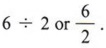2. Division is related to multiplication by the rule if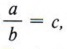then a = be. This is a check for all division problems. For instance, we know thatbecause 18 = (6)(3).
3. Division by zero is impossible. To evaluate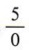we are required to find a number that, when multiplied by zero, will give 5. No such number exists.
4. A nonzero number divided by itself is 1.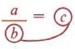. Multiply the circled quantities to obtain a. This is very important! If a is any nonzero number, then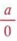has no meaning.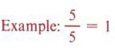From (3) we see that an expression such as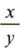is not meaningful unless we know that y ≠ 0. In this and future sections whenever we write a fraction it will be assumed that the denominator is not equal to zero. Now, to establish the division law of exponents, we will use the definition of exponents.

 Important! Read this paragraph again!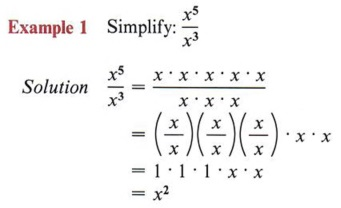We know that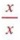= 1. We are also assuming that x represents a nonzero number.

In such an example we do not have to separate thequantities if we remember that a quantity divided by itself is equal to one. In the above example we could write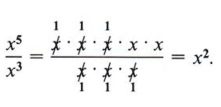Three x�s in the denominator will divide three x�s in the numerator.Remember that the 1 must be written if it is the only term in the numerator.

From the preceding examples we can generalize and arrive at the following law:

Third Law of Exponents If a and b are positive integers and x is a nonzero real number, then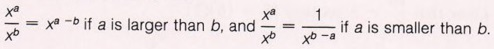If we try to use only the part of the law that states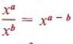on an expression such aswe would obtainAt this point negative exponents have not been defined. We will discuss them later.## DIVIDING A MONOMIAL BY A MONOMIAL

### OBJECTIVES

Upon completing this section you should be able to simplify an expression by reducing a fraction involving coefficients as well as using the third law of exponents.

We must remember that coefficients and exponents are controlled by different laws because they have different definitions. In division of monomials the coefficients are divided while the exponents are subtracted according to the division law of exponents.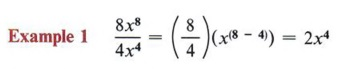If no division is possible or if only reducing a fraction is possible with the coefficients, this does not affect the use of the law of exponents for division.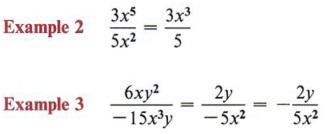Reduce this type of fraction in two steps: 1. Reduce the coefficients. 2. Use the third law of exponents.

## DIVIDING A POLYNOMIAL BY A MONOMIAL

### OBJECTIVES

Upon completing this section you should be able to divide a polynomial by a monomial.

To divide a polynomial by a monomial involves one very important fact in addition to things we already have used. That fact is this: When there are several terms in the numerator of a fraction, then each term must be divided by the denominator.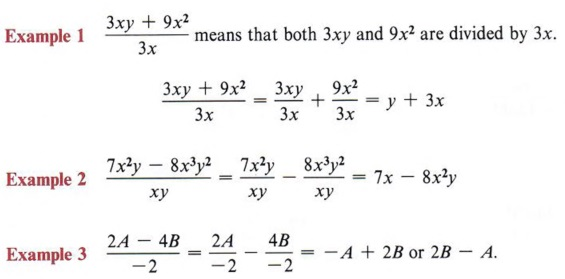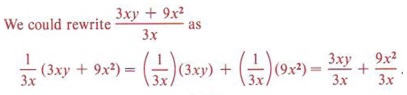Thus, we are actually using the distributive property in this process.

## DIVIDING A POLYNOMIAL BY A BINOMIAL

### OBJECTIVES

Upon completing this section you should be able to correctly apply the long division algorithm to divide a polynomial by a binomial.

The process for dividing a polynomial by another polynomial will be a valuable tool in later topics. Here we will develop the technique and discuss the reasons why it works in the future.

This technique is called the long division algorithm. An algorithm is simply a method that must be precisely followed. Therefore, we will present it in a step-by-step format and by example.

 Recall the three expressions in division: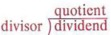If we are asked to arrange the expression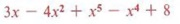in descending powers, we would write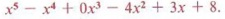. The coefficient zero gives 0x3 = 0. That is the reason the x3 term was missing or not written in the original expression.Solution

Step 1: Arrange both the divisor and dividend in descending powers of the variable (this means highest exponent first, next highest second, and so on) and supply a zero coefficient for any missing terms. (In this example the arrangement need not be changed and there are no missing terms.) Then arrange the divisor and dividend in the following manner: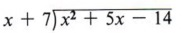Step 2: To obtain the first term of the quotient, divide the first term of the dividend by the first term of the divisor, in this case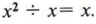. We record this as follows: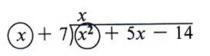Step 3: Multiply the entire divisor by the term obtained in step 2. Subtract the result from the dividend as follows: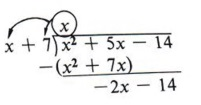Make sure you write the quotient directly over the quantity you are dividing into. In this case x divides into x2 x times.

Step 4: Divide the first term of the remainder by the first term of the divisor to obtain the next term of the quotient. Then multiply the entire divisor by the resulting term and subtract again as follows: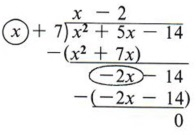The first term of the remainder (-2x - 14) is -2x. Multiply (x + 7) by -2.

This process is repeated until either the remainder is zero (as in this example) or the power of the first term of the remainder is less than the power of the first term of the divisor.

As in arithmetic, division is checked by multiplication. We must remember that (quotient) X (divisor) + (remainder) = (dividend).

To check this example we multiply (x + 7) and (x - 2) to obtain x2 + 5x - 14.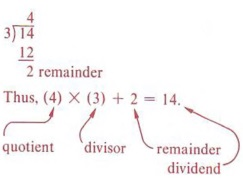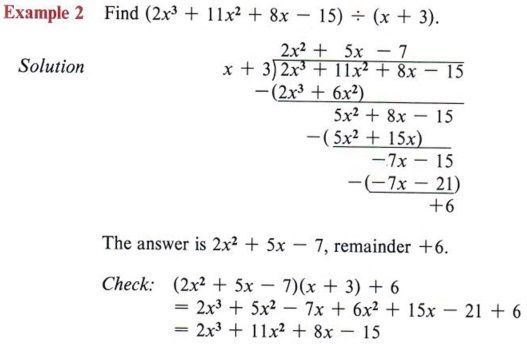Since this is the dividend, the answer is correct.

 Again, (quotient) X (divisor) + (remainder) = (dividend)The answer is x - 3. Checking, we find (x + 3)(x - 3)

 A common error is to forget to write the missing term with a zero coefficient.

## SUMMARY

### Key Words

• A monomial is an algebraic expression in which the literal numbers are related only by the operation of multiplication.
• A polynomial is the sum or difference of one or more monomials.
• A binomial is a polynomial having two terms.
• A trinomial is a polynomial having three terms.
• If x2 = y, then x is a square root of y.
• The principal square root of a positive number is the positive square root.
• The symbolis called a radical sign and indicates the principal square root of a number.
• A perfect square number has integers as its square roots.

### Procedures

• The first law of exponents is xaxb = xa+b.
• To find the product of two monomials multiply the numerical coefficients and apply the first law of exponents to the literal factors.
• To multiply a polynomial by another polynomial multiply each term of one polynomial by each term of the other and combine like terms.
• The second law of exponents is (xa)b = xab.
• The third law of exponents is• To divide a monomial by a monomial divide the numerical coefficients and use the third law of exponents for the literal numbers.
• To divide a polynomial by a monomial divide each term of the polynomial by the monomial.
• To divide a polynomial by a binomial use the long division algorithm.